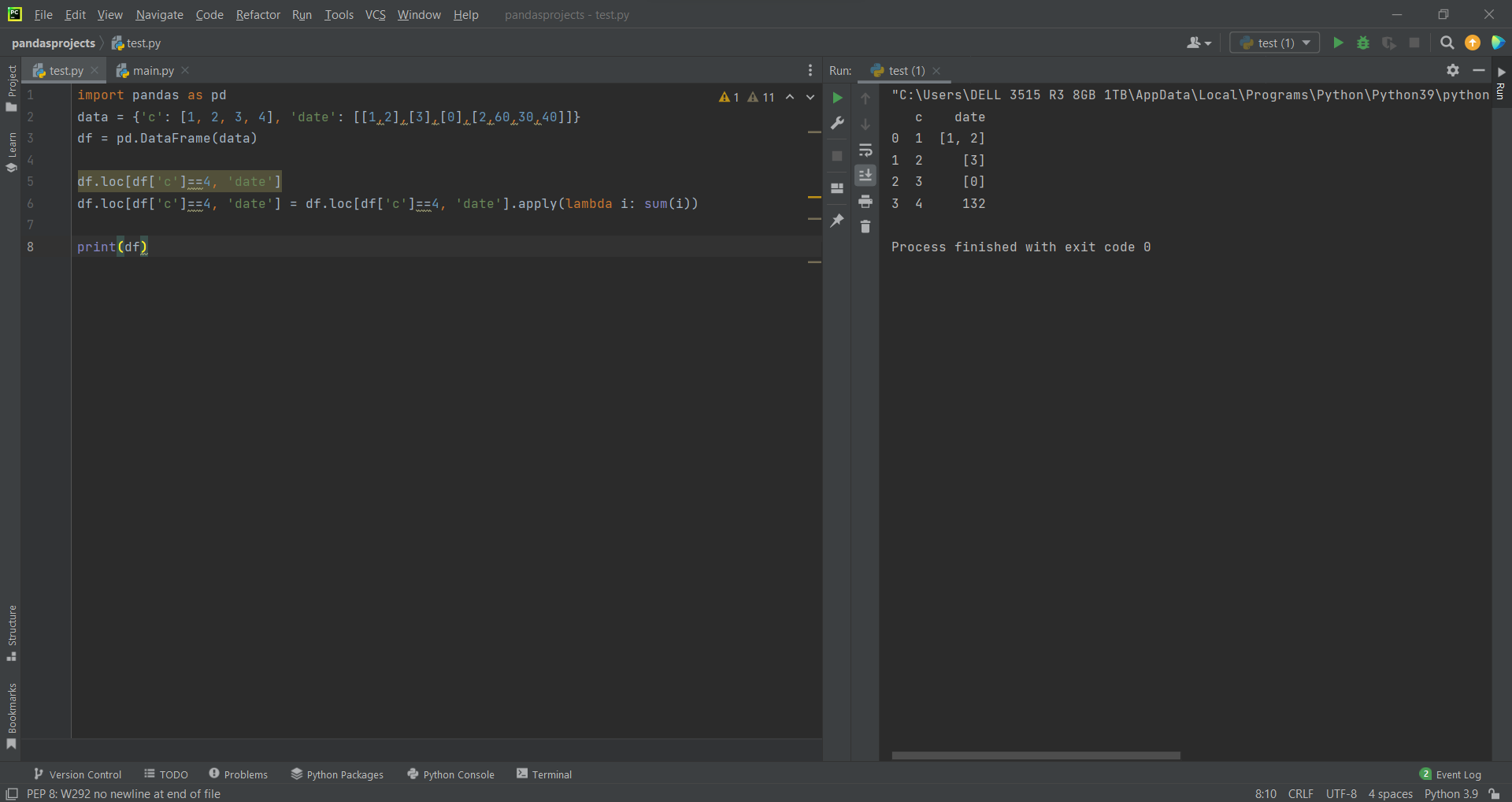# How to Create an Array in a Pandas DataFrameby Abdul Rawoof A RUpdated: Jan 31, 2023Solution Kit

An array in a DataFrame refers to a column of data with the same data type. A DataFrame can have multiple arrays, each representing a different data column. Each array in a DataFrame is a one-dimensional NumPy array, containing the values of that column. In pandas, you can create an array in a DataFrame in several ways:

• Using a list or array when creating the DataFrame.
• Expanding an existing DataFrame with a new column.
• Assigning a NumPy array to a new column.
• Using the “pd.concat()” function.
• pd.concat(): It is useful when combining data from multiple DataFrames into a single DataFrame for analysis or visualization.
• Using the pd.Series() function.
• pd.series(): pd.Series() is a function in the pandas library that creates a one-dimensional array-like object called a Series.
• Additionally, you may add a new column to a DataFrame and give it a value using the ".assign()" function or add a new column at a precise location using the ".insert()" method.

For better knowledge of arrays in DataFrame using Pandas, you may have a look at the code below.Fig : Preview of the output that you will get on running this code from your IDE.

### Code

In this solution we're using pandas library.

### Instructions

Follow the steps carefully to get the output easily.

2. Copy the snippet using the 'copy' and paste it in your IDE.
3. Add required dependencies and import them in Python file.
4. Run the file to generate the output.

I hope you found this useful. I have added the link to dependent libraries, version information in the following sections.

I found this code snippet by searching for 'Array in DataFrame using Panda' in kandi. You can try any such use case!

### Environment Tested

I tested this solution in the following versions. Be mindful of changes when working with other versions.

1. The solution is created in PyCharm 2021.3.
2. The solution is tested on Python 3.9.7.
3. Pandas version-v1.5.2.

Using this solution, we are able to implement array in dataframe using pandas with simple steps. This process also facilities an easy way to use, hassle-free method to create a hands-on working version of code which would help us to implement array in dataframe using pandas.

### Dependent Library

pandasby pandas-dev

Python38689Version:v2.0.2Flexible and powerful data analysis / manipulation library for Python, providing labeled data structures similar to R data.frame objects, statistical functions, and much more

Support
Quality
Security
Reuse

pandasby pandas-dev

Python38689Version:v2.0.2License: Permissive (BSD-3-Clause)

Flexible and powerful data analysis / manipulation library for Python, providing labeled data structures similar to R data.frame objects, statistical functions, and much more
Support
Quality
Security